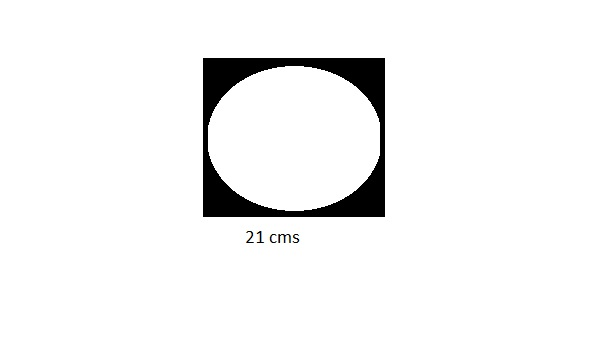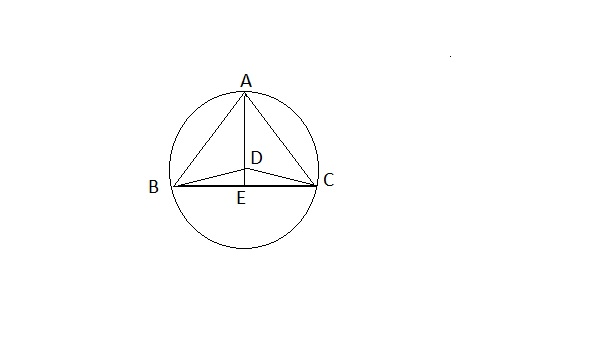0
276

Mensuration is an important topic in the Quant section of the SNAP Exam. Quant is a scoring section in SNAP, so it is advised to practice as much as questions from quant. This article provides some of the most important Mensuration Questions for SNAP. One can also download this Free Mensuration Questions for SNAP PDF with detailed answers by Cracku. These questions will help you practice and solve the Mensuration questions in the SNAP exam. Utilize this PDF practice set, which is one of the best sources for practicing.

Question 1: What is the area of the shaded portion, if the side of the square measure 21 ms.?a) 86.5 sq. cms.

b)

• 102 sq. cms.

c) 94.5 sq. cms.

d) 81.5 sq. cms.

Solution:

Area of shaded portion will be the area of square minus the area of the circle.

i.e

21^2 – (pi * 21^2 )/4

=94.5

Question 2: The area of the square of side 8 cm is equal to a rectangle. Which of the following statements/is/are definitely true about the rectangle (Sides of the rectangle are integers)?

a) The length of the rectangle is 16 times its breadth

b) The length of the rectangle is 32 times its breadth

c) The breadth of the rectangle is $\frac{1}{6}$ of its length

d) The breadth of the rectangle is $\frac{1}{9}$ of its length

e) None of these

Solution:

Area of rectangle = $8^{2}$.
Area of rectangle = 64 $cm^{2}$.
Length$\times$Breadth = 64.
Combination of Length and breadth is (32,2).
Hence, length of rectangle is 16 times the breadth of rectangle.
Therefore, Option A is correct.

Question 3: In the following figure, ABC is an equilateral triangle which is inscribed inside a circle and whose radius is r. Which of the following is the area of the triangle ?a) $(r-DE)^\frac{1}{2}(r+DE)^2$

b) $(r-DE)^2$ $(r+DE)^2$

c) $(r-DE)^\frac{1}{2}$ $(r+DE)^\frac{3}{2}$

d) $(r+DE)^2$ $(r-DE)^\frac{3}{2}$

e) None of these

Solution:BD=DC = r
According to Pythagoras Theorem , BD$^{2}$ = r$^{2}$ = DE$^{2}$+ BE$^{2}$
$BE^{2}$= $r$$^{2} – DE$$^{2}$
$BE$=$(r^{2} – DE^{2})$ $^{\frac{1}{2}}$
$r$$^{2} – DE$$^{2}$= $(r+DE) \times (r-DE)$.
$BC=2\times BE$=
$AE= (r+DE)$
Area of $\triangle$ ABC = $\frac{1}{2} \times BC \times AE$ = $\frac{1}{2} \times 2\times (r^{2}$ – $DE^{2})$ $\times$ $(r+DE)$
$\frac{1}{2} \times 2\times (r^{2}$ – $DE^{2})$ $\times$ $(r+DE)$ = $(r-DE) \times (r+DE) \times (r+DE)$ = $(r-DE)^\frac{1}{2}$ $(r+DE)^\frac{3}{2}$

Hence Option C is the correct answer.

Question 4: The radius of a circle is 20% more than the height of a right angled triangle. The base of triangle is 36 cm. If the area of triangle and circle be equal, what will be the area of circle ?

a) 72 $cm^2$

b) 144 $cm^2$

c) 216 $cm^2$

d) 128 $cm^2$

e) Cannot be determined

Solution:

Let the height of the triangle be ‘h’. Area of the triangle = (1/2) * 36 * h = 18h

Radius of the circle = 1.2h

Area of the circle = $\pi * 1.44 h^2$

Since the areas are equal, 18h = (22/7) * 1.44 * $h^2$

=> h = 18*7/1.44/22 = 3.97727273

So, the area of the circle = Area of the triangle = 18 * 3.97727273 = 72 $cm^2$ approx.

Question 5: The area of a rectangular field is 460 square metres.If the length is 15 per cent more than the breadth, what is breadth of the rectangular field ?

a) 15 metres

b) 26 metres

c) 34.5 metres

d) Cannot be determined

e) None of these

Solution:

let the length and breadth if rectangle be L and B respectively.

It is given that L = 1.15B

Area is given as 460 sqmtr

L x B = 460

So B = 20 m

Question 6: What will be the cost of gardening 1 metre broad boundary around a rectangular plot having perimeter of 340 meters at the rate of Rs 10 per square metre?

a) Rs 3,400/-

b) Rs 1,700/-

c) Rs 3,440/-

d) Cannot be determined

e) None of these

Solution:

here the cost of boundary making = 10 per sqmt

Total area to be boundaried = 2 L + 2B + (1×4)…(1)

It is given that perimeter of rectangle is 340 m

So 2(L + B ) = 340….(2)

From equation 1 and 2

Total area to be boundaried = 344 sq mtr

Cost of boundaring 344 sq mtr area = 344 × 10 = Rs 3440

Question 7: The area of a square is thrice the area of a rectangle If the area of the square is 225 sq cm and the length of the rectangle is 15 cm what is the difference between the breadth of the rectangle and the side of the square ?

a) 8 cm

b) 10 cm

c) 12 cm

d) 6 cm

e) None of these

Solution:

area of square = $(side)^2$ = 225

Side of square = 15 cm

It is given that area of square is thrice area of rectangle ,so

225 = 3 x L x B where L and B are length and breadth of rectangle.

Given that length of rectangle is 15 cm so B = 5 cm

Side of square – Breadth of rectangle = 15 -5 =10 cm

Question 8: The diameter of a circle is 7.7 cm What is the circumference of the circle ?

a) 26.4 cm

b) 24.2 cm

c) 28.4 cm

d) 22.2 cm

e) None of these

Solution:

diameter = 7.7 cm

Circumference = 2 π R = 2 x 3.14 x 3.85 = 24.178 cm ~24.2 cm

Question 9: A rectangular field has its length and breadth in the ratio of 6:5 respectively A man riding a bicycle completes one lap of this field along its perimeter at the speed of 19.8 km/h in 2 min What is the area of the field ?

a) 19200 sq m

b) 27000 sq m

c) 32500 sq m

d) Cannot be determined

e) None of these

Solution:

here the speed of cycle is given = 19.8 km/hr = 19.8 × $\frac{5}{18}$ m/s = 5.5 m/s

Time = 2 min = 120 sec

Distance = speed × time

Distance = 5.5 × 120 = 660 m

As length : breadth = 6:5

Length = 6y

Perimeter = 660 m

22y = 660

y = 30 m

So length = 180 m

Area = 180×150 = 27000 sq mtr

Question 10: What would be the area of a circle whose circumference is 35.2 cm?

a) 67.22 sq cm

b) 75.54 sq cm

c) 98.56 sq cm

d) 86.75 sq cm

e) None of these

Solution:

circumference of circle = 2πR = 35.2

R is the radius if circle

R= 5.6 cm

Area of circle = π$(R)^2$ = 3.14 × $(5.6)^2$ = 98.56 sq cm

Question 11: The diameter of a circle is 7.7 cm What is the circumference of the circle ?

a) 26.4 cm

b) 24.2 cm

c) 28.4 cm

d) 22.2 cm

e) None of these

Solution:

diameter = 7.7 cm

Circumference = 2 π R = 2 x 3.14 x 3.85 = 24.178 cm ~24.2 cm

Question 12: The area of a square is thrice the area of a rectangle If the area of the square is 225 sq cm and the length of the rectangle is 15 cm what is the difference between the breadth of the rectangle and the side of the square ?

a) 8 cm

b) 10 cm

c) 12 cm

d) 6 cm

e) None of these

Solution:

area of square = $(side)^2$ = 225

Side of square = 15 cm

It is given that area of square is thrice area of rectangle ,so

225 = 3 x L x B where L and B are length and breadth of rectangle.

Given that length of rectangle is 15 cm so B = 5 cm

Side of square – Breadth of rectangle = 15 -5 =10 cm

Question 13: What will be the cost of gardening 1 metre broad boundary around a rectangular plot having perimeter of 340 meters at the rate of Rs 10 per square metre?

a) Rs 3,400/-

b) Rs 1,700/-

c) Rs 3,440/-

d) Cannot be determined

e) None of these

Solution:

here the cost of boundary making = 10 per sqmt

Total area to be boundaried = 2 L + 2B + (1×4)…(1)

It is given that perimeter of rectangle is 340 m

So 2(L + B ) = 340….(2)

From equation 1 and 2

Total area to be boundaried = 344 sq mtr

Cost of boundaring 344 sq mtr area = 344 × 10 = Rs 3440

Question 14: The area of a rectangular field is 460 square metres.If the length is 15 per cent more than the breadth, what is breadth of the rectangular field ?

a) 15 metres

b) 26 metres

c) 34.5 metres

d) Cannot be determined

e) None of these